TitleIntermediate Algebra Tutorial 6: Practice Test on Tutorials 2 - 5

WTAMU > Virtual Math Lab > Intermediate AlgebraLearning Objectives

 After completing this tutorial, you should be able to: Take a test on topics covered in tutorials 2 - 5 in this website.

 Special Notes about Tutorial 6: I can not guarantee you will pass your test after going though any of the tutorials in this website or this practice test. However, it will definitely help you to better understand the topics covered better. Disclaimer: WTAMU and Kim Seward are not responsible for how a student does on any test or any class for any reason including not being able to access the website due to any technology problems.  There are no videos on this page.Introduction

It is important to note that, chances are, I'm not your math instructor. You need to check with your math teacher as to things like when your next math test is and what it covers.  It may cover more material on the test than what is in this practice test.  Just note that there are other practice tests at this website. So, after finding out what is on your test (if you have one) do the practice test(s) problems that go with the test you are preparing for.  If you are not in a class or are not having a test soon, this practice test is still good practice to go through and check to make sure you are understanding this material before moving on - kind of like a spot check.  The material on this practice test goes with Tutorial 2: Algebraic Expressions, Tutorial 3: Sets of Numbers, Tutorial 4: Operations on Real Numbers, and Tutorial 5: Properties of Real Numbers.

Also note that your teacher may word the problems on their test a little differently, may have some different kinds of problems, or may have a different number of problems than what is in this practice test.  Again, since I'm probably not your math instructor, I don't know exactly how your teacher will set up your math test.  Just note that these problems will help you build an understanding of the concepts presented and the terms used in math problems.  If you have an understanding of the problems instead of just memorizing them, then you should do fine on these concepts, no matter how the test is set up.

 Steps to Studying for a Math Test
1. Work through problems.  If you are in a class, you should have done this on completion of any homework you have done.  For anyone,  you can accomplish this by doing the practice problems found in each tutorial.

2. Check work on problems.  The practice problems in each tutorial have links to the answers to them so you can instantly check how you are doing.  Also, in most math books, the odd answers are found in the back of the book.

3. Review concepts.  Whether you got the problems right or wrong, make sure you review over them.  If you did get a problem wrong, make sure you either review that concept in it's respective tutorial or ask your math teacher about it.  If you don't ask about a problem before a test,  you are going to kick yourself when it comes up on the test.

4. Work through problems as if you were taking the test - no notes, book, webpages, etc.  This practice test is a perfect way to do that. After taking this practice test, check your answers by clicking on the link to the answer key found at the bottom of the practice test (before the 'need extra help on these topics' section)

 During the Test

It is to your benefit to show as much of the work as possible on the problems that have several steps involved.

Make sure that you read the directions carefully,  you wouldn't believe how many points get taken off  math tests for people not following directions.

Pace yourself.  You do not have to be the first one done to do well on the test.  Do not panic if there is still time left to take the test and others are turing it in.  Sometimes that means they do not know the material and left some of the answers blank.  Do not worry about anyone else but yourself.

Don't rush through a problem.  Another thing that math teachers take points off for are careless mistakes made by people that rush through a problem.  When those students get their tests back, they bonk themselves on the head at some of the things that got counted wrong,  things that they knew how to do.

Remember to breathe!!!!  I know some of you are scared to death at the thought of having to take a math test of any kind.  For you guys, try to relax and don't forget to breathe.  (Even if you aren't scared to take a math test,  it is probably a good idea to remember to breathe,  I wouldn't want you to pass out during the test). If it feels like your brain has left the building during your test, just close your eyes and breathe in and out and in and out and your brain will return.

Good luck on your test.  If you are taking a math test soon, don't panic, you are going to do great!!!Practice Test

 Problems 1a - 1b:  Find the value of the algebraic expression at  the given replacement values.

 1a.  x + y when   x = 5.2 and y = 3.9

 1b.  If it costs \$450 per tv at a local entertainment store, then we can use the algebraic expression 450x, where x represents the number of tv's purchased to find the cost of buying x tv's.  How much would it cost to buy 3?

 Problems 2a - 2b:  Write the phrase as an algebraic expression.

 2a.  The product of 12 and a number.

 2b.  The quotient of 25 and the difference of a number and 3.

 Problems 3a - 3c:  List the elements of each set.

 3a.  {x | x is a natural number between 10 and 20}

 3b.  {x | x is a whole number greater than 5}

 3c.  {x | x is a whole number less than 10}

 Problem 4a:  Graph the set on a number line.

 4a  {-3, -2.5, 0, 3/2, 3}

 Problems 5a - 5f :  List the elements of the following set that are also elements of the given set. {-5, -2.5, 0,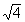,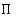(pi),}

 5a.  Natural numbers 5b.  Whole numbers

 5c.  Integers 5d.  Rational numbers

 5e.  Irrational numbers 5f.  Real numbers

 Problems 6a - 6b:  Placeor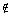to make each statement true.

 6a.  0 ? {x | x is a natural number}

 6b.   .25 ? {x | x is a rational number}

 Problems 7a - 7b:  Determine whether the statement is true or false.

 7a.7b.Problems 8a - 8c:  Find the absolute value.

 8a.   |4.2| 8b.  |- 4.2| 8c.  -|20|

 Problems 9a - 9b:   Find the sum or difference.

 9a.  -35 + (-15) 9b.  -14 - (-5)

 Problems 10a - 10b:  Find the product or quotient.

 10a.  (-15)(-10) 10b.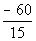Problems 11a - 11b:  Evaluate.

 11a.11b.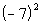Problems 12a - 12b:  Find the root.

 12a.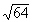12b.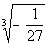Problems 13a - 13b: Simplify.

 13a.13b.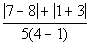Problem 14a: Find the value of the expression when a = 5 and b = -3.

 14a.   -6(2a - 3b)

 Problems 15a - 15b:  Write the opposite (additive inverse) and the reciprocal (multiplicative inverse) of each number.

 15a.  10 15b.   -1/2

 Problems 16a - 16b: Use a commutative property to write an equivalent expression.

 16a.  5 + b 16b.  7a

 Problems 17a - 17b:  Use an associative property to write an  equivalent expression.

 17a.  (-10a)b 17b.  x + (2y + 3z)

 Problems 18a - 18b:  Use the distributive property to find the product.

 18a.  -(5a - 6b) 18b.  3(2x + 4y + 5z)

 Problem 19a:   Simplify the expression.

 19a.  3(a - 4) + 4(a + 5)

 Problems 20a - 20b: Write each statement using mathematical symbols.

 20a.  The sum of x and 5 is less than or equal to the opposite of 9.

 20b.  Twice the difference of y and 6 is not equal to the reciprocal of 1/5.

 Problem 21a:   Write the following as an algebraic expression.

 21a.   The cost of x hamburgers, if each hamburger costs \$1.75.

 Problems 22a - 22c:  Insert <, > , or = to form a true statement.

 22a.  1.5   ?  3/2 22b.  -3    ?   -1 22c.   0   ?   -5

 Now you are ready to check the answers to your practice test:Need Extra Help on these Topics?

The following are web pages that can assist you in the topics that were covered on this page:

 Tutorial 2:  Algebraic Expressions This tutorial will help you with problems 1a - 1b and 2a - 2b on this practice test. Tutorial 3: Sets of Numbers This tutorial will help you with problems 3a - 3c, 4a, 5a - 5f, 6a - 6b, 7a - 7b and 8a - 8c on this practice test. Tutorial 4: Operations on Real Numbers This tutorial will help you with problems 9a - 9b, 10a - 10b, 11a - 11b, 12a- 12b, 13a - 13b and 14a -14b on this practice test. Tutorial 5: Properties of Real Numbers This tutorial will help you with problems 15a - 15b, 16a - 16b, 17a - 17b, 18a - 18b, 19a, 20a - 20b, 21a, and 22a - 22c on this practice test.

Go to Get Help Outside the Classroom found in Tutorial 1: How to Succeed in a Math Class for some more suggestions.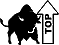Last revised on June 17, 2011 by Kim Seward.
All contents copyright (C) 2002 - 2011, WTAMU and Kim Seward. All rights reserved.